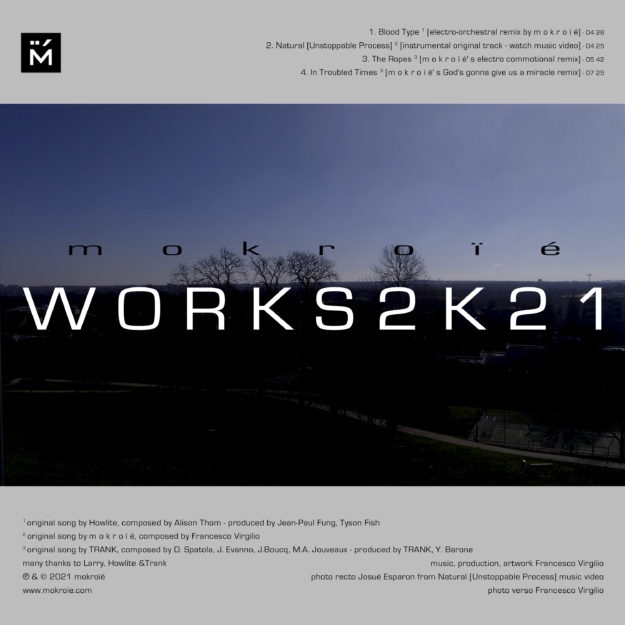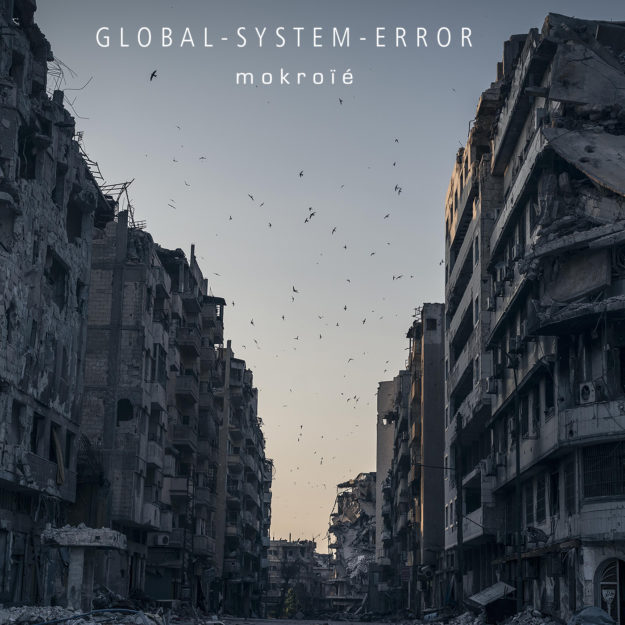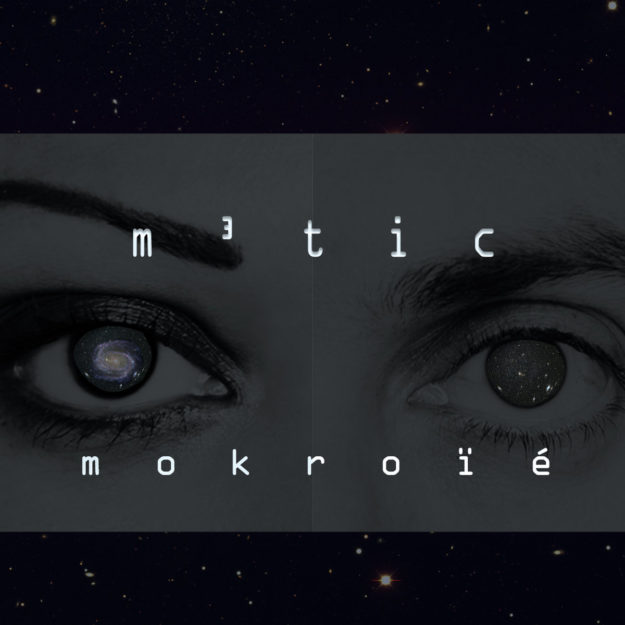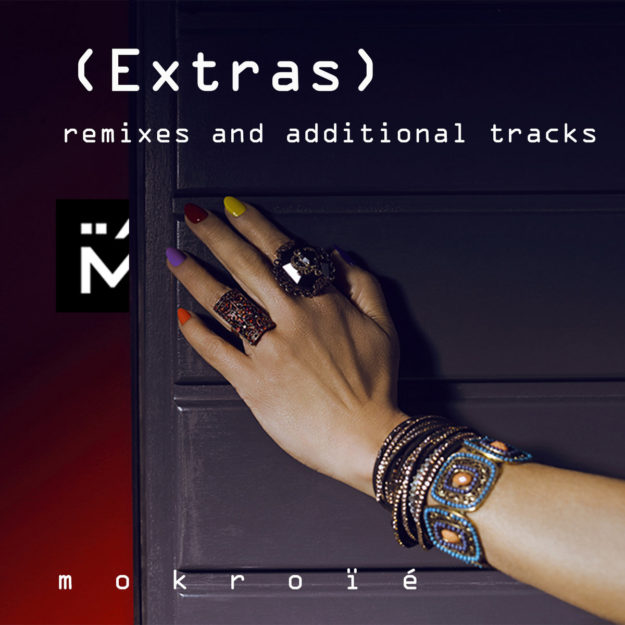###### W O R K S 2 K 2 1###### G L O B A L – S Y S T E M – E R R O R###### m​ ³ ​t i c###### T H E  T R I A L###### ( E X T R A S )R e c a p  D I S C O G R A P H Y

W O R K S 2 K 2 1

M A C H I N E S  &  S O U L

G L O B A L – S Y S T E M – E R R O R

m³tic [ micro macro mokroïé’s travels in colors ]

T H E  T R I A L

( E x t r a s ) – remixes and additional tracks

B A N D C A M P